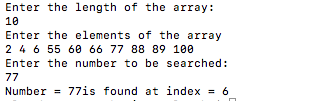# Jump Search Implementation using C++

In this article, we are going to learn what is Jump search, and how to implement it using C++ program?
Submitted by Aleesha Ali, on February 08, 2018

Jump search is a Searching algorithm and its efficiency lies between linear search and binary search.

Here, we search or find a particular element with the help of step size means we search a particular element by jumping or skipping the in-between values according to the step size given by the user if a particular element is still not found then this algorithm will follow simple linear search on previous elements.

For applying this​​ algorithm elements must be in sorted order means ascending or descending.

Complexity:

```
sqrt(n) or (√n).
Sqrt = SquareRoot.

```

## Implementation of Jump Search using C++

```//Implementation of jump search using c++

#include <iostream>
#include <vector>
#include <cmath>

using namespace std;

int jumpSearchProg(vector <int> arr, int noToSearch, int ArrayLim)
{
int previous = 0;
int step = sqrt(ArrayLim);
//Step to skip elements for jumping

while (arr[min(step,ArrayLim)-1] < noToSearch)
{
previous = step;
step += sqrt(ArrayLim);
if(previous >= ArrayLim) return -1;
}

/*Applying linear Search and starting from the previous elements*/
while (arr[previous] < noToSearch)
{
previous++;
/*If element has not found yet then it means element is not present in the array*/
if (previous == min(step, ArrayLim)) return -1;
}
// if we found the element then

if (arr[previous] == noToSearch)
return previous;

return -1;
}

//Start of main
int main()
{
int n,NoToSr;
cout<<"Enter the length of the array:"<<endl;
cin>>n;
vector<int> arr(n);

cout<<"Enter the elements of the array"<<endl;
for(int i=0;i<n;i++)
{
cin>>arr[i];
}

cout<<"Enter the number to be searched:"<<endl;
cin>>NoToSr;

//function calling
int result = jumpSearchProg(arr, NoToSr, n);

//displayin foud number
cout<<"Number = "<<NoToSr<<"is found at index = "<<result<<endl;
return 0;
}
```

OutputWhat's New

Top Interview Coding Problems/Challenges!

Languages: » C » C++ » C++ STL » Java » Data Structure » C#.Net » Android » Kotlin » SQL
Web Technologies: » PHP » Python » JavaScript » CSS » Ajax » Node.js » Web programming/HTML
Solved programs: » C » C++ » DS » Java » C#
Aptitude que. & ans.: » C » C++ » Java » DBMS
Interview que. & ans.: » C » Embedded C » Java » SEO » HR
CS Subjects: » CS Basics » O.S. » Networks » DBMS » Embedded Systems » Cloud Computing
» Machine learning » CS Organizations » Linux » DOS
More: » Articles » Puzzles » News/Updates